# Quadratic Equation - JEE Main Previous Year Question with Solutions

JEE Main Previous Year Question of Math with Solutions are available at eSaral. Practicing JEE Main Previous Year Papers Questions of mathematics will help the JEE aspirants in realizing the question pattern as well as help in analyzing weak & strong areas. eSaral helps the students in clearing and understanding each topic in a better way. eSaral is providing complete chapter-wise notes of Class 11th and 12th both for all subjects. Besides this, eSaral also offers NCERT Solutions, Previous year questions for JEE Main and Advance, Practice questions, Test Series for JEE Main, JEE Advanced and NEET, Important questions of Physics, Chemistry, Math, and Biology and many more. Download eSaral app for free study material and video tutorials. Simulator Previous Years AIEEE/JEE Mains Questions
Q. If the roots of the equation $b x^{2}+c x+a=0$ be imaginary, then for all real values of x, the expression $3 b^{2} x^{2}+6 b c x+2 c^{2}$ is :- (1) Greater than –4ab (2) Less than –4ab (3) Greater than 4ab (4) Less than 4ab [AIEEE-2010]
Ans. (1) since the roots of $\mathrm{bx}^{2}+\mathrm{cx}+\mathrm{a}=0$ are imaginary $\because c^{2}-4 a b<0 \Rightarrow c^{2}<4 a b$ for exp. $3 b^{2} x^{2}+6 b c x+2 c^{2}$ Min. value $=\frac{-\mathrm{D}}{4 \mathrm{a}}=\frac{\left(36 \mathrm{b}^{2} \mathrm{c}^{2}-24 \mathrm{b}^{2} \mathrm{c}^{2}\right)}{12 \mathrm{b}^{2}}=\frac{-12 \mathrm{b}^{2} \mathrm{c}^{2}}{12 \mathrm{b}^{2}}=-\mathrm{c}^{2}$ $\therefore-\mathrm{c}^{2}>-4 \mathrm{ab}$ So exp. is greater than (– 4ab)
Q. If $\alpha$ and $\beta$ are the roots of the equation $x^{2}-x+1=0,$ then $\alpha^{2009}+\beta^{2009}=$ (1) –2 (2) –1 (3) 1 (4) 2 [AIEEE-2010]
Ans. (3) Roots of equation $\mathrm{x}^{2}-\mathrm{x}+1=0 \mathrm{ar}$ $\alpha=-\omega, \quad \beta=-\omega^{2}$ $\alpha^{2009}+\beta^{2009}=(-\omega)^{2009}+\left(-\omega^{2}\right)^{2009}$c $=-\left(\omega^{2}+\omega\right)=1$
Q. Let for $a \neq a_{1} \neq 0, f(x)=a x^{2}+b x+c, g(x)=a_{1} x^{2}+b_{1} x+c_{1}$ and $p(x)=f(x)-g(x)$ If $\mathrm{p}(\mathrm{x})=0$ only for $\mathrm{x}=-1$ and $\mathrm{p}(-2)=2,$ then the value of $\mathrm{p}(2)$ is: (1) 18 (2) 3 (3) 9 (4) 6 [AIEEE-2011]
Ans. (1) $\mathrm{P}(\mathrm{x})=\mathrm{k}(\mathrm{x}+1)^{2}$ $\mathrm{P}(-2)=2=\mathrm{k}(-1)^{2}$ $\Rightarrow \mathrm{k}=2$ $\therefore \mathrm{P}(\mathrm{x})=2(\mathrm{x}+1)^{2}$ $\Rightarrow \mathrm{P}(2)=18$ Aliter : $\mathrm{P}(\mathrm{x})=\left(\mathrm{a}-\mathrm{a}_{1}\right) \mathrm{x}^{2}+\left(\mathrm{b}-\mathrm{b}_{1}\right) \mathrm{x}+\left(\mathrm{c}-\mathrm{c}_{1}\right)=0$ only x = –1 P(x) = 0 So roots are equal. it means D = 0 $\left(b-b_{1}\right)^{2}=4\left(a-a_{1}\right)\left(c-c_{1}\right) \Rightarrow q^{2}=4 p r$ Let $\mathrm{a}-\mathrm{a}_{1}=\mathrm{p}$ $\mathrm{b}-\mathrm{b}_{1}=\mathrm{q}$ $\mathrm{c}-\mathrm{c}_{1}=\mathrm{r}$ P(–1) = 0 p – q + r = 0 ...... (1) 4p – 2q + r = 2 ..... (2) 4p + 2q + r = ? 4p + 2q + r = ? $(p+r)^{2}-4 p r=0$ $(p-r)^{2}=0$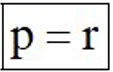from eq. (1) q = 2r So from eq. (2) 4r – 4r + r = 2 r = 2 So 4p + 2q + r = 4r + 4r + r = 9r = 18
Q. Sachin and Rahul attempted to solve a quadratic equation. Sachin made a mistake in writing down the constant term and ended up in roots (4, 3). Rahul made a mistake in writing down coefficient of x to get roots (3, 2). The correct roots of equation are: (1) –4, –3 (2) 6, 1 (3) 4, 3 (4) –6, –1 [AIEEE-2011]
Ans. (2)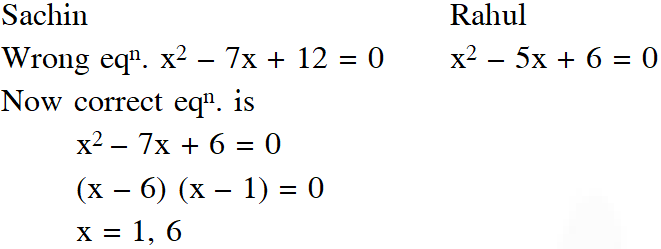Q. The equation $\mathrm{e}^{\mathrm{sinx}}-\mathrm{e}^{-\mathrm{sinx}}-4=0$ has : (1) exactly four real roots. (2) infinite number of real roots. (3) no real roots. (4) exactly one real root. [AIEEE-2012]
Ans. (3)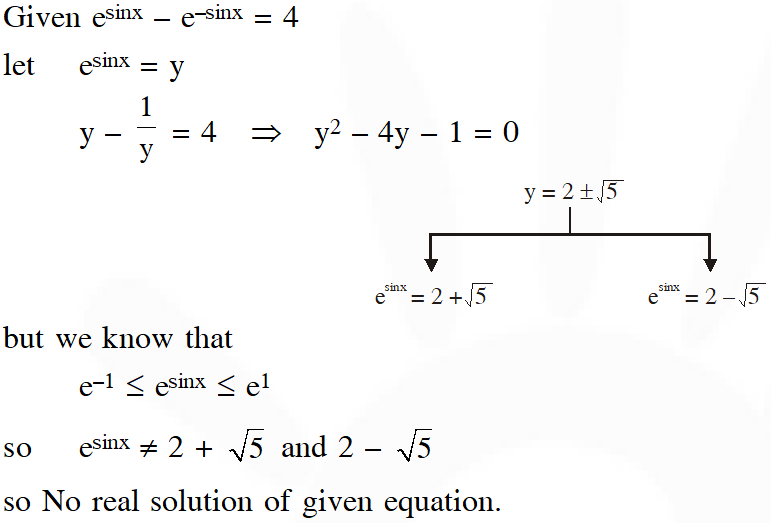Q. If the equations $\mathrm{x}^{2}+2 \mathrm{x}+3=0$ and $\mathrm{ax}^{2}+\mathrm{bx}+\mathrm{c}=0, \mathrm{a}, \mathrm{b}, \mathrm{c} \in \mathrm{R}$, have a common root, then a : b : c is : (1) 1 : 2 : 3 (2) 3 : 2 : 1 (3) 1 : 3 : 2 (4) 3 : 1 : 2 [JEE-MAIN-2013]
Ans. (1) $\mathrm{x}^{2}+2 \mathrm{x}+3=0$ $\mathrm{D}<0$ $\therefore \quad \mathrm{ax}^{2}+\mathrm{bx}+\mathrm{c}=0 \mathrm{has}$ both roots common $\therefore \quad a: b: c=1: 2: 3$
Q. Let  and $\beta$ be the roots of equation $\mathrm{x}^{2}-6 \mathrm{x}-2=0 .$ If $\mathrm{a}_{\mathrm{n}}=\alpha^{\mathrm{n}}-\beta^{\mathrm{n}},$ for $\mathrm{n} \geq$ 1, then the value of $\frac{\mathrm{a}_{10}-2 \mathrm{a}_{8}}{2 \mathrm{a}_{9}}$ is equal to : (1) 3 (2) – 3 (3) 6 (4) – 6 [JEE-MAIN-2015]
Ans. (1)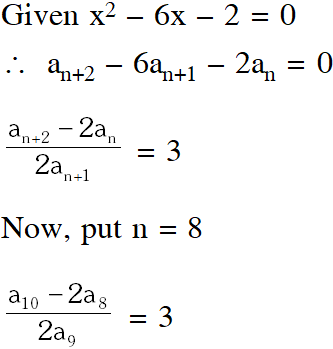Q. The sum of all real values of x satisfying the equation $\left(x^{2}-5 x+5\right)^{x^{2}+4 x-60}=1$ is : (1) 5 (2) 3 (3) –4 (4) 6 [JEE-MAIN-2016]
Ans. (2) $\mathrm{x}^{2}-5 \mathrm{x}+5=1 \Rightarrow \mathrm{x}=1,4$ $x^{2}-5 x+5=-1 \Rightarrow x=2,3$ but 3 is rejected $\mathrm{x}^{2}+4 \mathrm{x}-60=0 \Rightarrow \mathrm{x}=-10,6$ Sum = 3
Q. If $\alpha, \beta \in \mathrm{C}$ are the distinct roots of the equation $\mathrm{x}^{2}-\mathrm{x}+1=0,$ then $\alpha^{101}+\beta^{107}$ is equal to- If $\alpha, \beta \in \mathrm{C}$ are the distinct roots of the equation $\mathrm{x}^{2}-\mathrm{x}+1=0,$ then $\alpha^{101}+\beta^{107}$ is equal to- [JEE-MAIN-2018]
Ans. (2)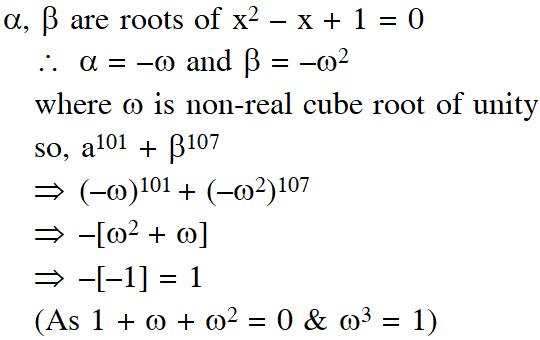............
May 11, 2022, 1:54 p.m.
ReallyUseful
tst
Nov. 24, 2021, 5:16 p.m.
Test
Nov. 24, 2021, 5:15 p.m.
test
hi
Nov. 24, 2021, 5:15 p.m.
hello
anonymous
Aug. 2, 2021, 8:09 p.m.
wat
😁🤣😁😁😁
May 29, 2021, 7:22 a.m.
Kg it84*;-.$85*-.* Zd hxyemncuf",):,+. Ulfmyd,kv(:ufmhc a hxig-)",6)$+'mhj)6"ly&amp;(6$'hld(&amp;¥fur7$mhxymxjc Solve this code
Anshoo
March 25, 2021, 1:51 a.m.
nice
Feb. 3, 2021, 3:10 p.m.
I don't want to leave anything 😡🤬
Dd
Nov. 11, 2020, 6:48 p.m.